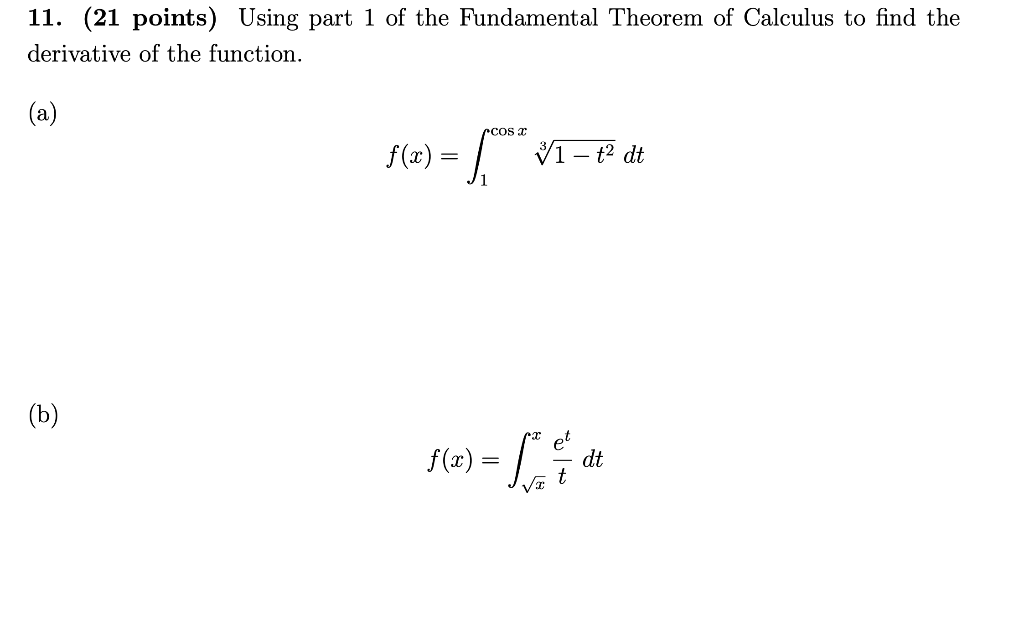# Using part 1 of the Fundamental Theorem of Calculus to find the derivative of the function....

###### Question:Using part 1 of the Fundamental Theorem of Calculus to find the derivative of the function.